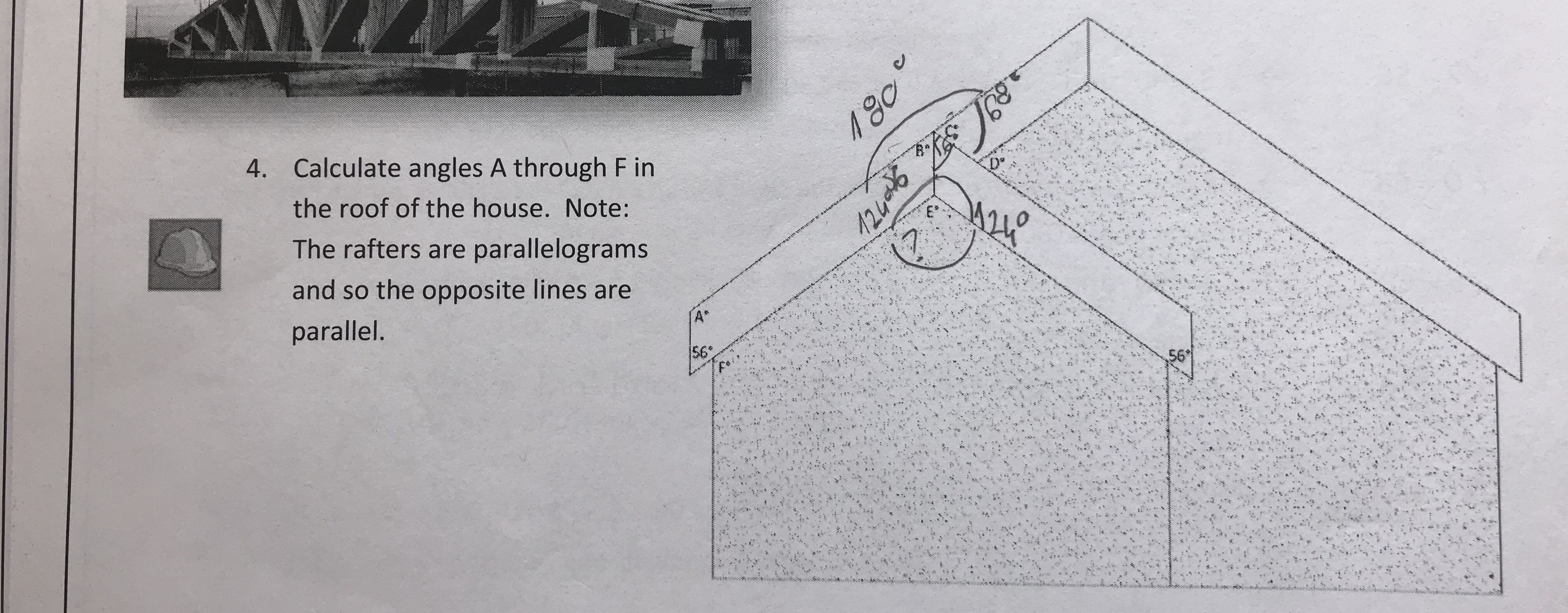# Calculate angles A through F inthe roof of the house. Note:The rafters are parallelogramsand so the opposite lines areparallel4.5656

Question

Please provide detailed explanation, easy to understand.help_outlineImage TranscriptioncloseCalculate angles A through F in the roof of the house. Note: The rafters are parallelograms and so the opposite lines are parallel 4. 56 56 fullscreen
check_circle

star
star
star
star
star
1 Rating
Step 1

To calculate the angles through in the roof of the house.

From figure, it is observed that the rafters are parallelograms and so the opposite lines are parallel.

label the figure as follows.

Step 2

From figure, PQRS and RSTU are parallelograms.

It is known that, the opposite angles of the parallelogram are equal.

Therefore, = 56° and  ∠TSR = 56°.

It is known that, the consecutive angles of the parallelogram are supplementary.

Therefore, A°+56° = 180°.

Step 3

The angle is calcula...

### Want to see the full answer?

See Solution

#### Want to see this answer and more?

Solutions are written by subject experts who are available 24/7. Questions are typically answered within 1 hour.*

See Solution
*Response times may vary by subject and question.
Tagged in

### Mensuration